Constructing bisector of angle and perpendicular bisector

Chapter 11 Class 9 Constructions
Concept wise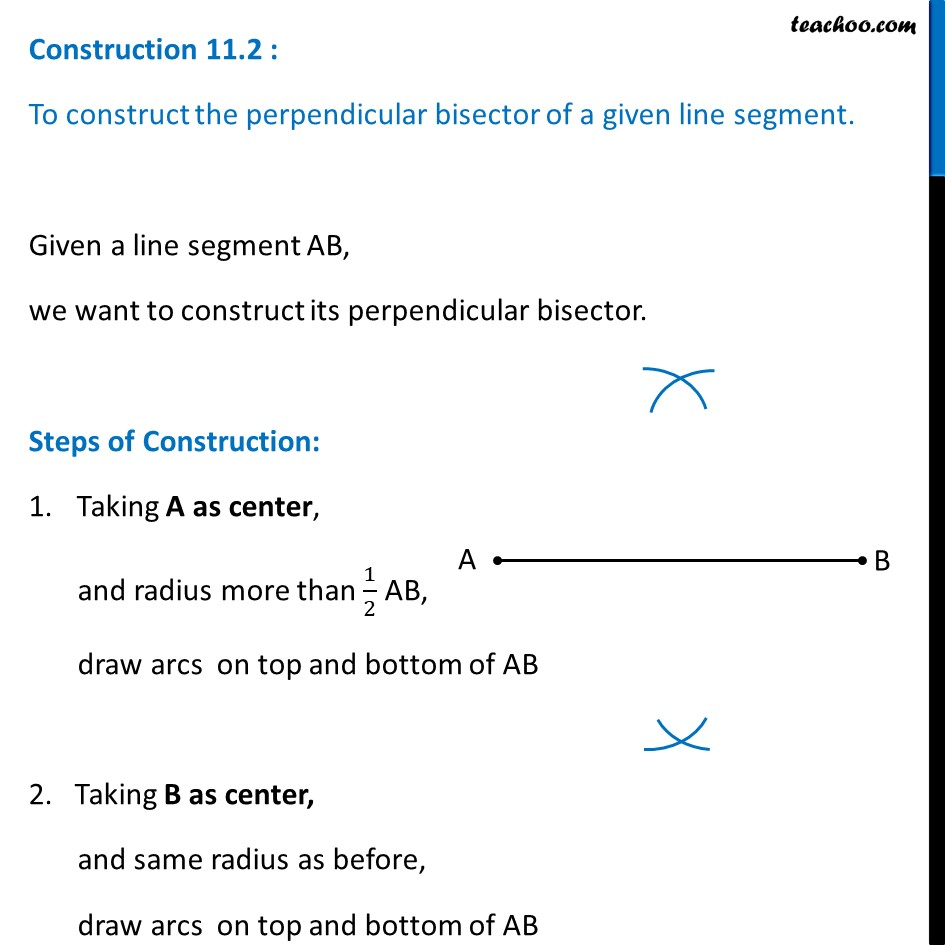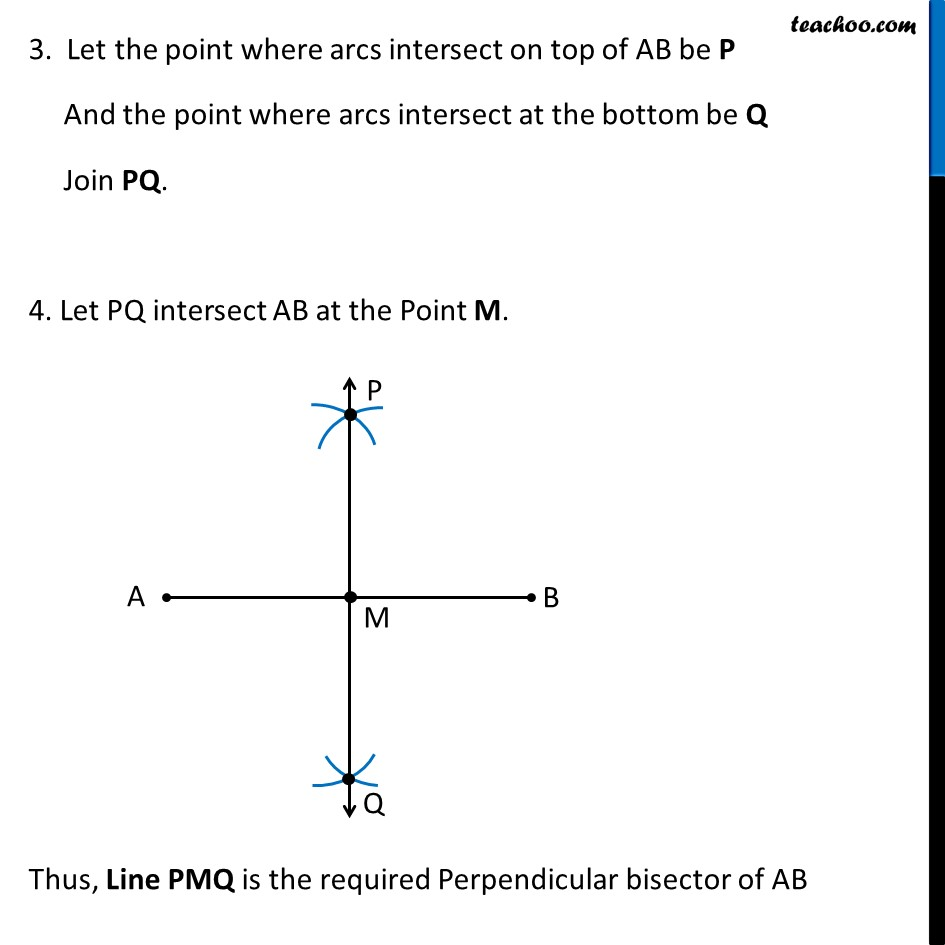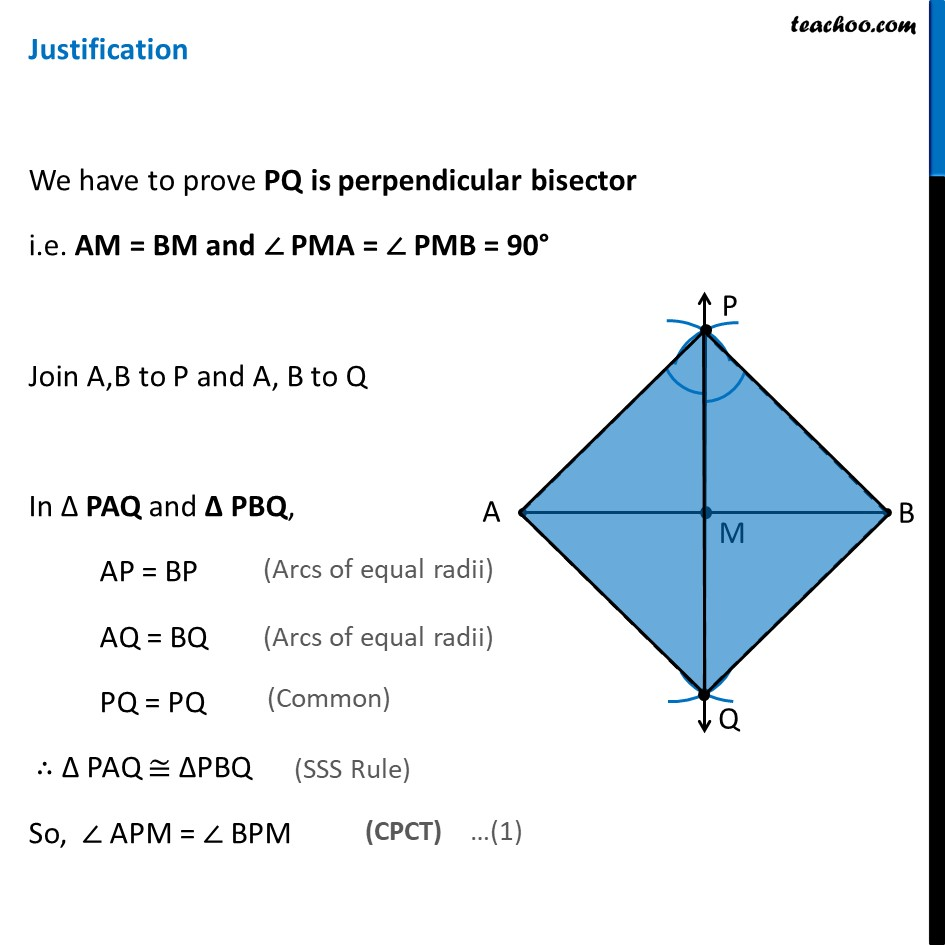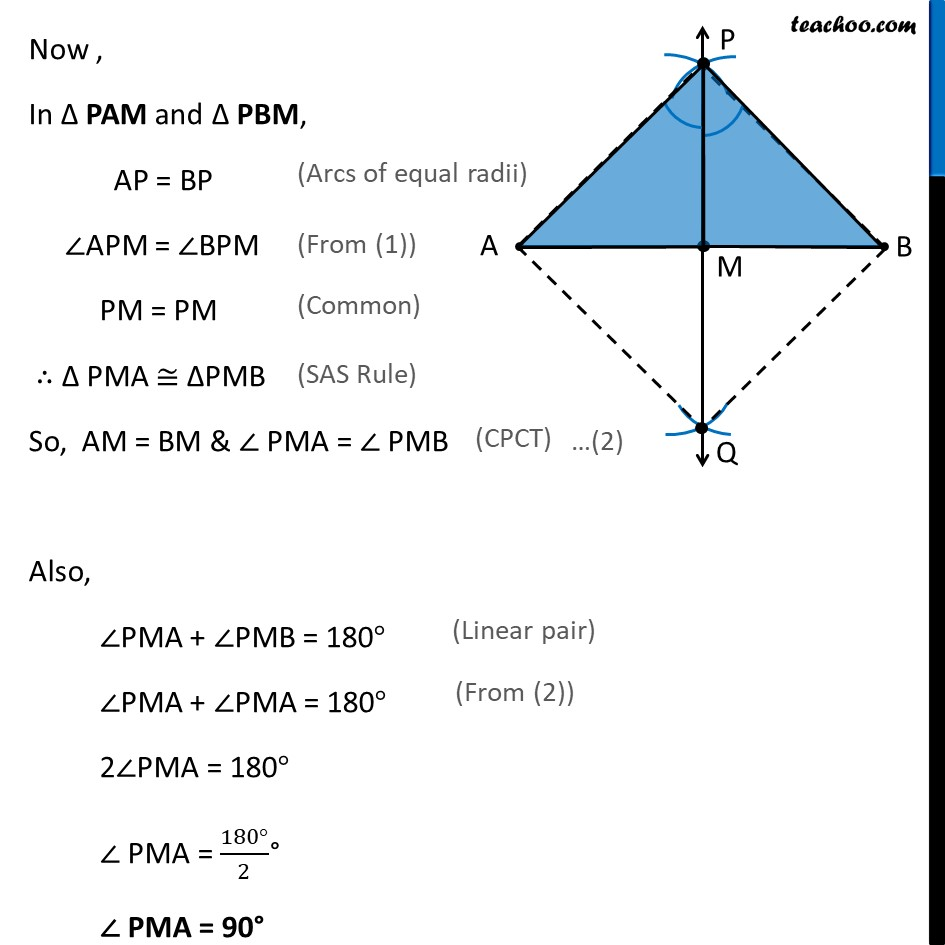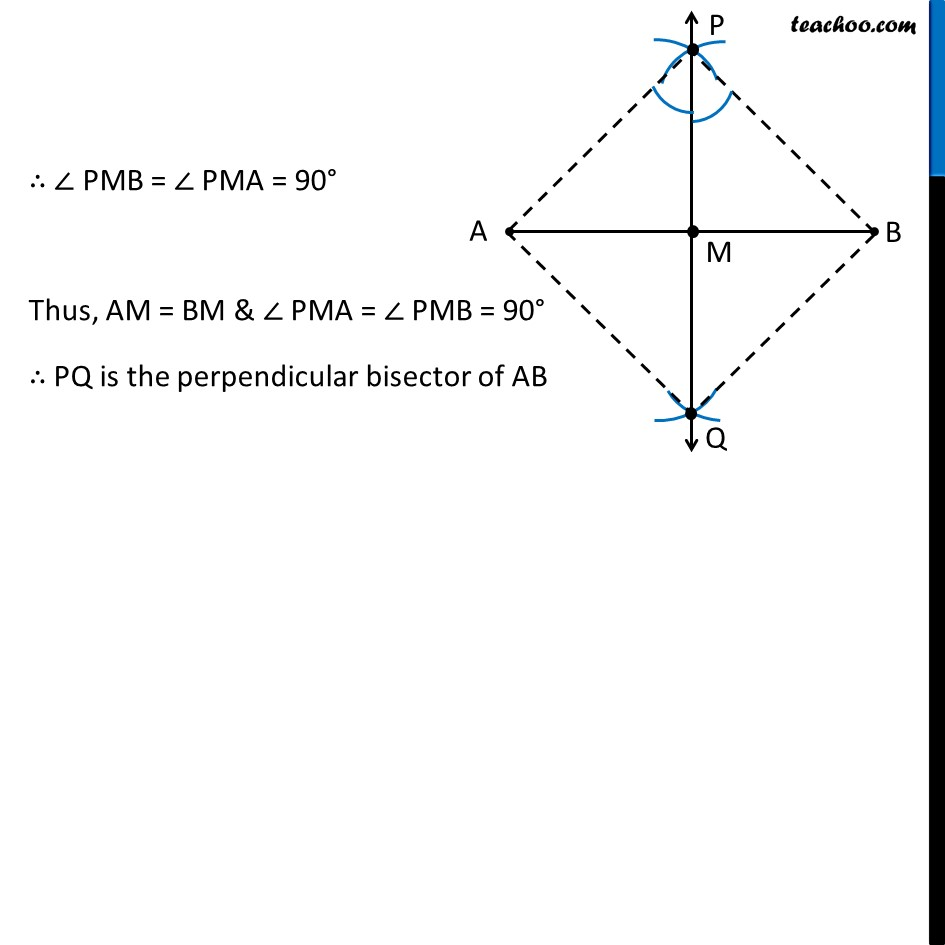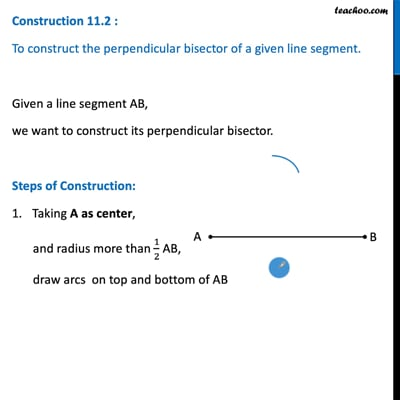This video is only available for Teachoo black users

Introducing your new favourite teacher - Teachoo Black, at only ₹83 per month

### Transcript

Construction 11.2 : To construct the perpendicular bisector of a given line segment. Given a line segment AB, we want to construct its perpendicular bisector. Steps of Construction: Taking A as center, and radius more than 1/2 AB, draw arcs on top and bottom of AB 2. Taking B as center, and same radius as before, draw arcs on top and bottom of AB 3. Let the point where arcs intersect on top of AB be P And the point where arcs intersect at the bottom be Q Join PQ. 4. Let PQ intersect AB at the Point M. Thus, Line PMQ is the required Perpendicular bisector of AB Justification We have to prove PQ is perpendicular bisector i.e. AM = BM and ∠ PMA = ∠ PMB = 90° Join A,B to P and A, B to Q In Δ PAQ and Δ PBQ, AP = BP AQ = BQ PQ = PQ ∴ ∆ PAQ ≅ ∆PBQ So, ∠ APM = ∠ BPM (Arcs of equal radii) (Arcs of equal radii) (Common) (SSS Rule) (CPCT) Now , In Δ PAM and Δ PBM, AP = BP ∠APM = ∠BPM PM = PM ∴ ∆ PMA ≅ ∆PMB So, AM = BM & ∠ PMA = ∠ PMB Also, ∠PMA + ∠PMB = 180° ∠PMA + ∠PMA = 180° 2∠PMA = 180° ∠ PMA = (180°)/2° ∠ PMA = 90° (Arcs of equal radii) (From (1)) (Common) (SAS Rule) (Linear pair) (From (2)) ∴ ∠ PMB = ∠ PMA = 90° Thus, AM = BM & ∠ PMA = ∠ PMB = 90° ∴ PQ is the perpendicular bisector of AB